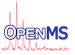OpenMS  2.8.0
LocalLinearMap Class Reference

Trained Local Linear Map (LLM) model for peak intensity prediction. More...

`#include <OpenMS/ANALYSIS/PIP/LocalLinearMap.h>`

Collaboration diagram for LocalLinearMap:
[legend]

## Classes

struct  LLMParam
Define parameters needed by the Local Linear Map (LLM) model. More...

## Public Member Functions

LocalLinearMap ()
default constructor More...

virtual ~LocalLinearMap ()
destructor More...

const LLMParamgetLLMParam () const
return parameters of the LocalLinearMap model More...

const Matrix< double > & getCodebooks () const
return position of the codebook vectors (18-dim) More...

const Matrix< double > & getMatrixA () const
return linear mappings of the codebooks More...

const std::vector< double > & getVectorWout () const
return linear bias More...

const Matrix< UInt > & getCord () const
return coordinates of codebook vectors on the 2-d grid More...

void normalizeVector (std::vector< double > &aaIndexVariables)
calculate and return normalized amino acid index variables from string representation of peptide More...

std::vector< double > neigh (const Matrix< UInt > &cord, Size win, double radius)
calculate neighborhood function based on distance of prototypes to winner prototype on two-dimensional grid structure and neighborhood width. More...

## Private Member Functions

Matrix< UIntgenCord_ (Size xdim, Size ydim)
needed to store prototype coordinates More...

double dist_ (const Matrix< UInt > &u, const Matrix< UInt > &v, Size a, Size b)
calculate distance between two prototypes More...

LocalLinearMap (LocalLinearMap &rhs)
Copy constructor not implemented => private. More...

LocalLinearMapoperator= (const LocalLinearMap &llm)
Assignment operator not implemented => private. More...

## Private Attributes

LLMParam param_
parameters of the model More...

Matrix< double > code_
codebook vectors More...

Matrix< double > A_
linear mappings More...

std::vector< double > wout_
linear bias More...

Matrix< UIntcord_
coordinates of codebooks on grid More...

## Detailed Description

Trained Local Linear Map (LLM) model for peak intensity prediction.

This class offers a model for predictions of peptide peak heights (referred to as intensities) by a Local Linear Map (LLM) model and is the basis of PeakIntensityPredictor.

A general introduction to the Peak Intensity Predictor (PIP) can be found in the PIP Tutorial.

The model trained needs two files for storing the position of the codebook vectors and the linear mappings (codebooks.data, linearMapping.data) This is the default model used by PeakIntensityPredictor.

## ◆ OpenMS::LocalLinearMap::LLMParam

 struct OpenMS::LocalLinearMap::LLMParam

Define parameters needed by the Local Linear Map (LLM) model.

Parameters xdim and ydim define the size of the two dimensional grid structure. Parameter radius gives the width of the Gaussian neighborhood function.

Collaboration diagram for LocalLinearMap::LLMParam:
[legend]
Class Members

width of Gaussian neighborhood function

UInt xdim

size of first coordinate

UInt ydim

size of second coordinate

## ◆ LocalLinearMap() [1/2]

 LocalLinearMap ( )

default constructor

## ◆ ~LocalLinearMap()

 virtual ~LocalLinearMap ( )
virtual

destructor

## ◆ LocalLinearMap() [2/2]

 LocalLinearMap ( LocalLinearMap & rhs )
private

Copy constructor not implemented => private.

## ◆ dist_()

 double dist_ ( const Matrix< UInt > & u, const Matrix< UInt > & v, Size a, Size b )
private

calculate distance between two prototypes

## ◆ genCord_()

 Matrix genCord_ ( Size xdim, Size ydim )
private

needed to store prototype coordinates

## ◆ getCodebooks()

 const Matrix& getCodebooks ( ) const

return position of the codebook vectors (18-dim)

## ◆ getCord()

 const Matrix& getCord ( ) const

return coordinates of codebook vectors on the 2-d grid

## ◆ getLLMParam()

 const LLMParam& getLLMParam ( ) const

return parameters of the LocalLinearMap model

## ◆ getMatrixA()

 const Matrix& getMatrixA ( ) const

return linear mappings of the codebooks

## ◆ getVectorWout()

 const std::vector& getVectorWout ( ) const

return linear bias

## ◆ neigh()

 std::vector neigh ( const Matrix< UInt > & cord, Size win, double radius )

calculate neighborhood function based on distance of prototypes to winner prototype on two-dimensional grid structure and neighborhood width.

## ◆ normalizeVector()

 void normalizeVector ( std::vector< double > & aaIndexVariables )

calculate and return normalized amino acid index variables from string representation of peptide

## ◆ operator=()

 LocalLinearMap& operator= ( const LocalLinearMap & llm )
private

Assignment operator not implemented => private.

## ◆ A_

 Matrix A_
private

linear mappings

## ◆ code_

 Matrix code_
private

codebook vectors

## ◆ cord_

 Matrix cord_
private

coordinates of codebooks on grid

## ◆ param_

 LLMParam param_
private

parameters of the model

## ◆ wout_

 std::vector wout_
private

linear bias